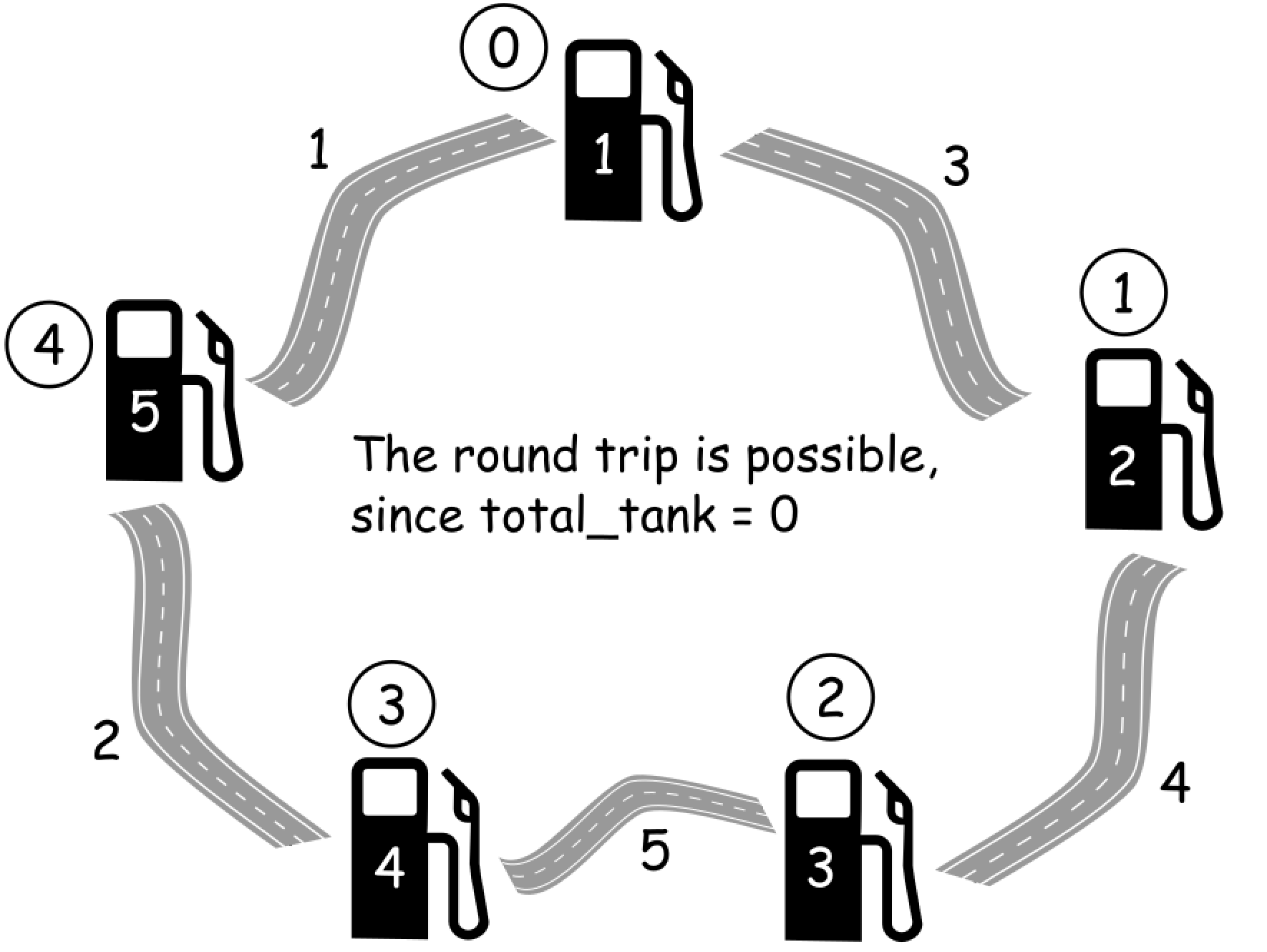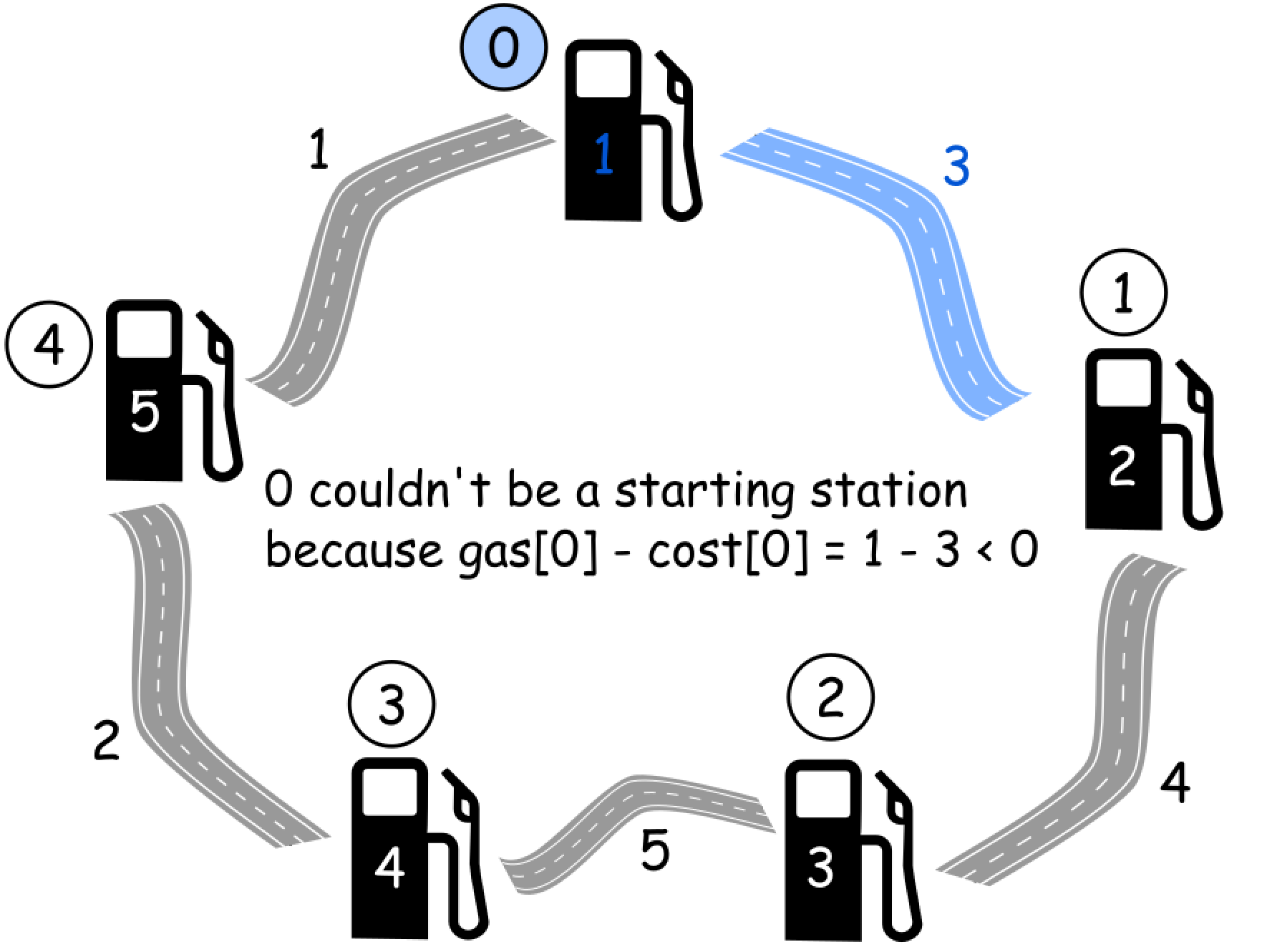# 【LeetCode】14 加油站

## 题目：

• 如果题目有解，该答案即为唯一答案。
• 输入数组均为非空数组，且长度相同。
• 输入数组中的元素均为非负数。

输入:
gas  = [1,2,3,4,5]
cost = [3,4,5,1,2]



输入:
gas  = [2,3,4]
cost = [3,4,3]



## 方法：一次遍历

• 选择该加油站为出发站
• 模拟汽车环路行驶，在每一个加油站检查我们还剩多少升汽油。1、初始化 total_tankcurr_tank为 0 ，并且选择 0 号加油站为起点。

2、遍历所有的加油站：

• 每一步中，都通过加上 gas[i]和减去cost[i] 来更新 total_tankcurr_tank

• 如果在 i + 1号加油站，curr_tank < 0 ，将 i + 1号加油站作为新的起点，同时重置 curr_tank = 0 ，让油箱也清空。

3、如果total_tank < 0，返回-1，否则返回 starting station## 代码

package com.janeroad;

public class test4 {
public int canCompleteCircuit(int[] gas, int[] cost) {
int n = gas.length;
int total_tank = 0;//总油量
int curr_tank = 0;//当前油量
int starting_station = 0;//起始站
for (int i = 0; i < n; ++i) {
total_tank += gas[i] - cost[i];//总油量=总油量+第i个加油站加油量-第i段公路耗油量
curr_tank += gas[i] - cost[i];//当前油量=当前油量+第i个加油站加油量-第i段公路耗油量
// 如果当前油量<0，就代表加的油不够走当前这段公路
if (curr_tank < 0) {
// 所以当前这个加油站不能作为起始站，故 起始站+1
starting_station = i + 1;
// 当前油量重新置0，进入下一层遍历继续判断
curr_tank = 0;
}
}
//遍历的最后，如果总油量>=0，说明会有一个加油站能够作为起始站，使得汽车走完整个环形公路，返回起始站变量值
//否则返回-1
}

public static void main(String[] args) {
test4 test4=new test4();
int[] gas= {1,2,3,4,5};//每一个加油站的油量
int[] cost={3,4,5,1,2};//每一段公路的耗油量
System.out.println( test4.canCompleteCircuit(gas,cost));
}

}